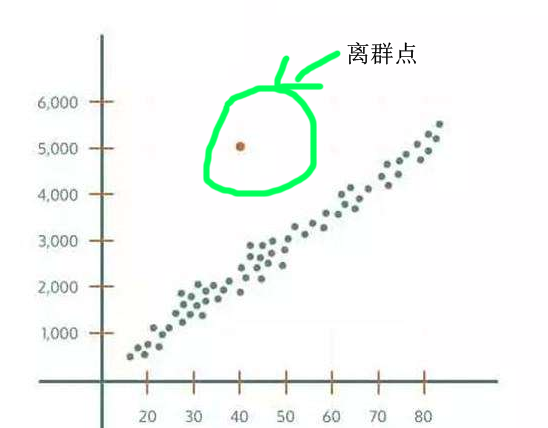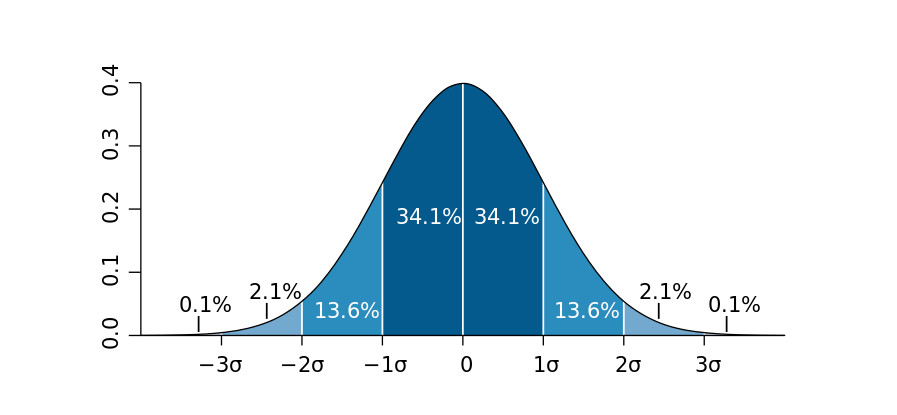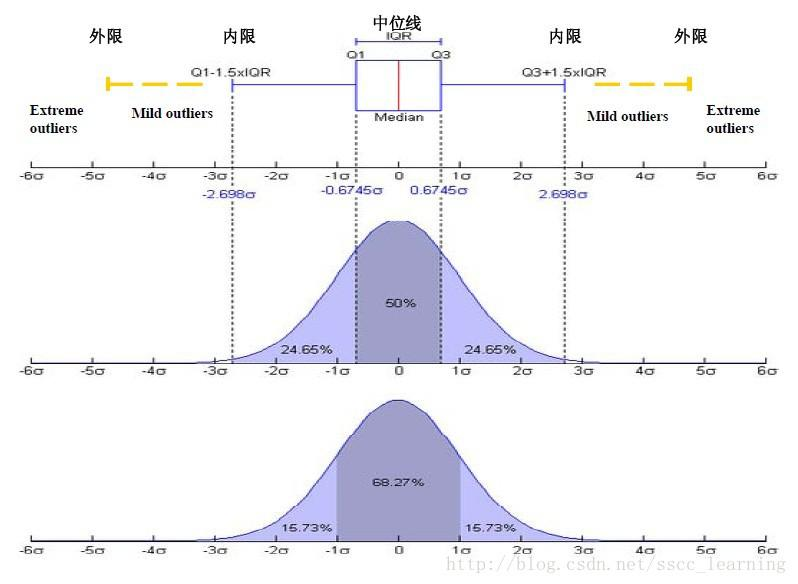# 机器学习实战-数据预处理(异常值处理)

## 什么样的值是异常值？## 判别方法：

### 2. 3δ原则### 3．箱型图分析## 异常值的处理方法常用有四种：

1.删除含有异常值的记录

2.将异常值视为缺失值，交给缺失值处理方法来处理

3.用平均值来修正

4.不处理

## 代码实现

（1）箱型图

import pandas as pd
import numpy as np
from collections import Counter

def detect_outliers(df,n, features):

outlier_indices = []

for col in features:
Q1 = np.percentile(df[col], 25)
Q3 = np.percentile(df[col], 75)

IQR = Q3 - Q1

outlier_step = 1.5 * IQR

outlier_list_col = df[(df[col] < Q1 - outlier_step) | (df[col] > Q3 + outlier_step)].index

outlier_indices.extend(outlier_list_col)

outlier_indices = Counter(outlier_indices)
multiple_outliers = list( k for k, v in outlier_indices.items() if v > n)

return multiple_outliers

df = df.drop(Outliers_to_drop, axis=0).reset_index(drop=True)